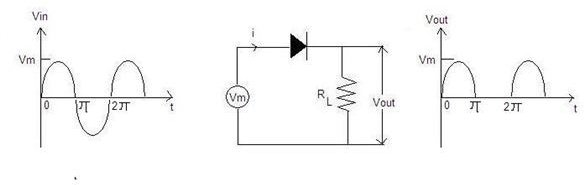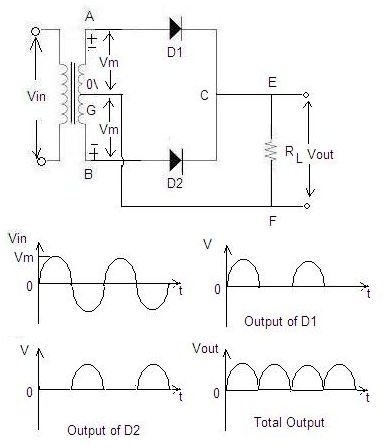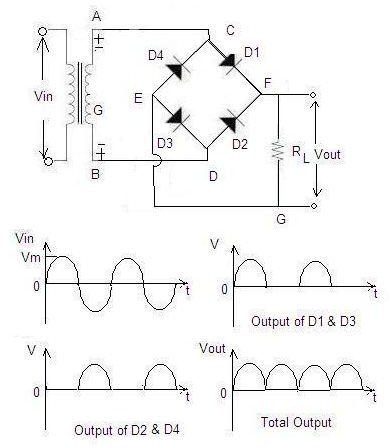# AC Rectifier Efficiency

## Types of Rectifier Circuits

A rectifier is the device used to do this conversion.

Rectifiers are of two types: half-wave rectifiers and full-wave rectifiers. Full-wave rectifiers are further classified as center tap full-wave rectifiers and bridge rectifiers.Half-wave Rectifier

The basic half-wave rectifier circuit and the input and output waveforms are shown in the diagram. A half-wave rectifier conducts only during the positive half cycle. It allows only one half of an AC waveform to pass through the load, RL, hence, the name half-wave rectifier. During the positive half cycle, the diode is forward biased and will act as a short circuit, allowing the waveform to pass through the load. During the negative half cycle, the diode is reversed biased and will act as an open circuit.

Efficiency of the Half-wave Rectifier

Rectifier efficiency is defined as the ratio of DC power to the applied input AC power.

Rectifier efficiency, η = DC output power/input AC power

Idc= Im / π

The DC output power is given by: Pdc= I2dc x RL = (Im / π)2 x RL

The AC input power is given by: Pac= I2rms(rf + RL) , where rf is diode resistance.

For a half-wave rectified wave: Irms= Im / 2

Therefore, Pac=(Im/2)2 x (rf+RL)

Thus rectifier efficiency, η = DC output power/AC input power

= [(Im/π)2xRL] / [(Im/2)2x(rf+RL)]

= 0.406 RL/ (rf + RL)

= 0.406 / (1+rf/RL)

The efficiency will be maximum if rf is negligible as compared to RL

Therefore, maximum rectifier efficiency = 40.6%. This means only 40.6% of the input AC power is converted into DC power.

## Full-Wave Rectifier

Center Tap Full-Wave RectifierA center tap full-wave rectifier circuit with two diodes and a transformer is shown in the diagram. The center tap is taken as the ground or zero voltage reference point. Unlike the half-wave rectifier, both the positive as well as the negative half cycles of the input are used with the help of two diodes and a center tapped transformer. During the positive half cycle of the AC input, terminal A will be positive and terminal B will be negative. Therefore, diode D1 will be forward biased and diode D2 will be reversed biased. The current will flow along AD1CEFG. The positive half cycle will appear across RL as shown in second waveform.

During the negative half cycle, the terminal B becomes positive and terminal A becomes negative. Diode D1 will be reversed biased and diode D2 will be forward biased. The current will flow along BD2CEFG.

Idc= 2 Im /π = 2 Vm / π RL

Irms= Im / √2 = 0.707 Im

Rectifier Efficiency, η = Pdc / Pac

= I2dcRL/ I2rms (rf + RL)

= 0.812 / [1+ (rf / RL)]

= 81.2% if the diode resistance rf is negligible as compare to RL.

The efficiency of the center tap full-wave rectifier is twice the value of the half-wave rectifier.

## Bridge RectifierThe bridge rectifier is the most commonly used circuit for DC power supplies. It consists of four diodes D1 through D4, as shown in the diagram, connected to form a bridge. During the positive input half cycle, terminal A will be positive and terminal B will be negative. Diodes D1 and D3 will become forward biased and D2 and D4 will be reversed biased. The current will flow along ACFGEDB producing a drop across load resistance RL. During the negative input half cycle, the terminal B will be positive and terminal A will be negative. Diodes D2 and D4 will become forward biased and diodes D1 and D3 will become reversed biased. The current will flow along BDFGECA. Current flows through load resistance RL in the same direction FG during both AC input half cycles.

Bridge rectifier efficiency, η = Pdc / Pac

= 81.2% (calculated using the same formulas used for the center tap full-wave rectifier)

This means that a full-wave bridge rectifier is also twice as efficient as a half-wave rectifier.

## References and Images

Images created by author.

References

Basic Electronics by B.L.Theraja, Chand (S.) & Co Ltd., India 2007 (Paperback)

Bright Hub, Inc.: How to Build a Bridge Rectifier, Swagatam Majumdar

Micronotes (Microsemi Scottsdale): Rectifier Bridges and Dual Rectifiers, Kent Walters (PDF)